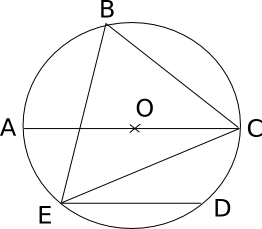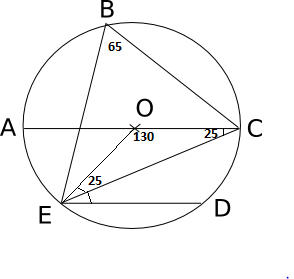Question 29

#In the adjoining figure, chord ED is parallel to the diameter AC of the circle. If angle CBE = 65°, then what is the value of angle DEC?

SolutionIf EBC = 65 then EOC = 130 then OEC = OCE = 25. NOw since OC and ED are parallel we have OCE = OED = 25. Hence option D.